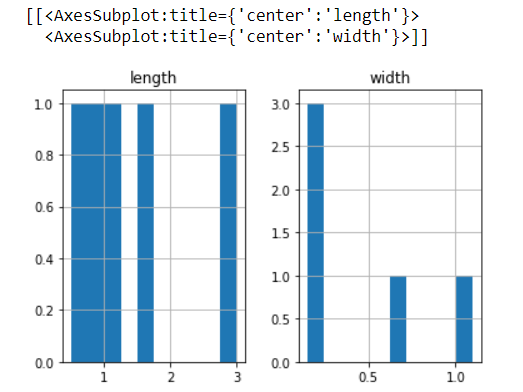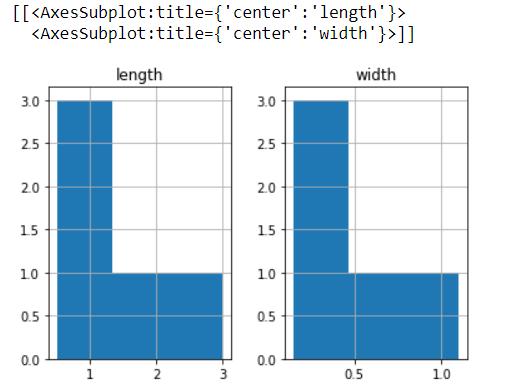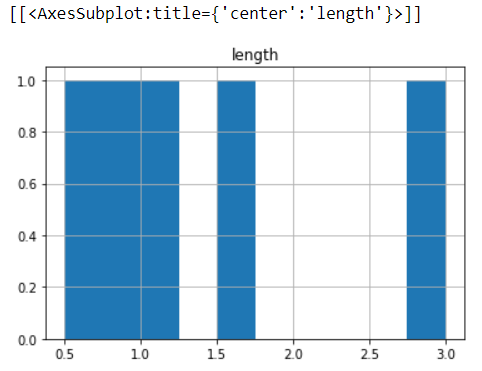Dark Mode On/Off

# Pandas DataFrame hist() Method

To get the summarized data in a visual representation, we use the histogram and in this tutorial, we will learn the Python pandas `DataFrame.hist()` method. This method makes a histogram of the DataFrame’s. A histogram can be defined as the representation of the distribution of data. This method calls `matplotlib.pyplot.hist()`, on each series in the DataFrame, resulting in one histogram per column.

The below is the syntax of the `DataFrame.hist()` method.

### Syntax

``DataFrame.hist(column=None, by=None, grid=True, xlabelsize=None, xrot=None, ylabelsize=None, yrot=None, ax=None, sharex=False, sharey=False, figsize=None, layout=None, bins=10, backend=None, legend=False, **kwargs)``

### Some of the important parameters of `DataFrame.hist()` method are,

data: It is the DataFrame that the pandas object holding the data.

column: It represents the str or sequence. If this parameter is passed, it will be used to limit data to a subset of columns.

by: It represents the object, optional. If this parameter is passed, then used to form histograms for separate groups.

grid: It represents the bool(True or False), and the default is True. It indicates whether to show axis grid lines.

bins: It is the int or sequence, and the default value is 10 and it represents the number of histogram bins to be used. If we give an integer, bins + 1 bin edges are calculated and returned. If we give bins as a sequence, it gives bin edges, which includes the left edge of the first bin and the right edge of the last bin. In this case, bins are returned unmodified.

## Example: Creating histograms of columns of the DataFrame using the `DataFrame.hist()` method

In the below example, we created a DataFrame, and let's apply the `DataFrame.floordiv()` method to the DataFrame and get the visual representation of the DataFrame. We will get the graph based on the DataFrame columns that are the length and width. See the below example.

``````#importing pandas as pd
import pandas as pd
df = pd.DataFrame({'length': [1.5, 0.5, 1.2, 0.9, 3],'width': [0.7, 0.2, 0.15, 0.2, 1.1]}, index=['python', 'C', 'C++', 'R', 'Pearl'])
print(df.hist())``````## Example: Creating histograms of columns of the DataFrame using the `DataFrame.hist()` method with bins method.

The `DataFrame.hist()` method draws a histogram based on the length and width of some indexes, displayed in three bins. See the below example.

``````#importing pandas as pd
import pandas as pd
df = pd.DataFrame({'length': [1.5, 0.5, 1.2, 0.9, 3],'width': [0.7, 0.2, 0.15, 0.2, 1.1]},index=['python', 'C', 'C++', 'R', 'Pearl'])
print(df.hist(bins=3))``````## Example: Set column='length' in the `DataFrame.hist()` method

Here, we gave the column as length and the `DataFrame.hist()` method draws a histogram based on the specified column. See the below example.

``````#importing pandas as pd
import pandas as pd
df = pd.DataFrame({'length': [1.5, 0.5, 1.2, 0.9, 3],'width': [0.7, 0.2, 0.15, 0.2, 1.1]},index=['python', 'C', 'C++', 'R', 'Pearl'])
print(df.hist(column="length"))``````### Conclusion:

In this tutorial, we learned the Python pandas `DataFrame.hist()` method. We learned the syntax and by applying this function on the DataFrame we solved examples and understood the `DataFrame.hist()` method.

Want to learn coding?
Try our new interactive courses.
Over 20,000+ students enrolled.